# Tree - (Recursion|Induction) Algorithm

A recursive algorithms invoke themselves as a subroutine with a smaller input.

The idea of the recursion tree method is to write out all of the work done by the recursive algorithm in a tree structure, with the children of a given node corresponding to the recursive calls made by that node.

The relationship between the elements is called a Recurrence relation

## Base case

Recursive algorithms need a base case so they don't keep calling themselves until the rest of time. When the input's sufficient (small), you stop the recursion and you just return some trivial answer.

## Tree definiton

The tree can be:

### Tree Function

Example: defining a sequence of number by recursion Ref. A tree function is known as a recurrence relation and is a function that expresses each element of a sequence as a function of the preceding ones.

``````seq = ();
def recurenceRelation(x):
if (x <10){
recurenceRelation(x+1)
}```
```

## Documentation / Reference

Discover More(Tree|Nested Set|Hierarchy) Data Structure

A tree is a node that may have children. Tree's are inherently recursive by definition as each child of a node is a Tree itself, with or without children nodes. A tree is a special case of a graph structure...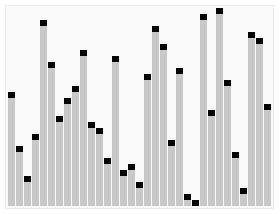Algorithm - Divide-and-Conquer algorithm design paradigm

You take a problem, and break it down into smaller sub problems which you then solve recursively and then you somehow combine the results of the smaller sub-problem to get a solution to the original problem...Code - Grammar / Syntax (Lexical)

This section regroups the entity of a computer language from a lexical point of view. It's the same as Parts of the speech for a natural language. Grammars are useful models when designing software...File System - File System Tree Traversal

Because a file system arranges file in a tree structure (file system tree), when you want to read it, you perform a which is therefore a recursion Pseudo codeInteger - Multiplication (Product)

Integer multiplication Input: 2 n digit numbers x and y where n is large in the thousands or even more Output: the product x times y Primitive Operation (unit of performance): add or multiply...
Javascript - Function Expression (Anonymous function)

A Reference/Operators/functionFunction Expression is just an function in the form of an expression stored generally in a variable that you execute by adding its signature ([param,[, param,[..., param]]])...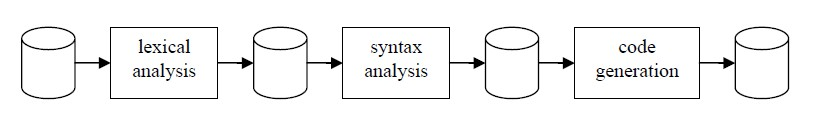Lexical Analysis - Parser (Syntax analysis|Linter)

A parser create a parse tree data structure from a series of token created by the lexer. The creation of the tree is based on the rules declared in the grammar (which defines the syntactic structure of...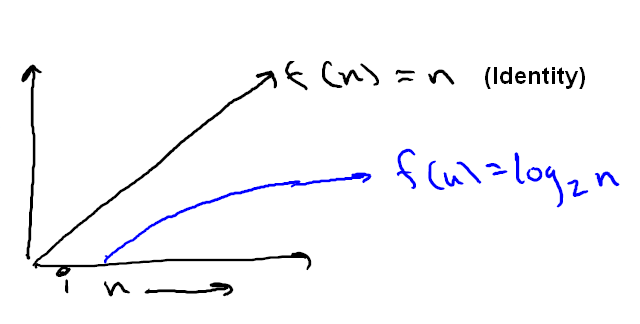Mathematics - Logarithm Function (log)

is the number (#) of times you divide n by b until you get down to 1. trees With the base 2 where is the # of times you divide n by 2 until you get down to 1. You keep repeating dividing by two...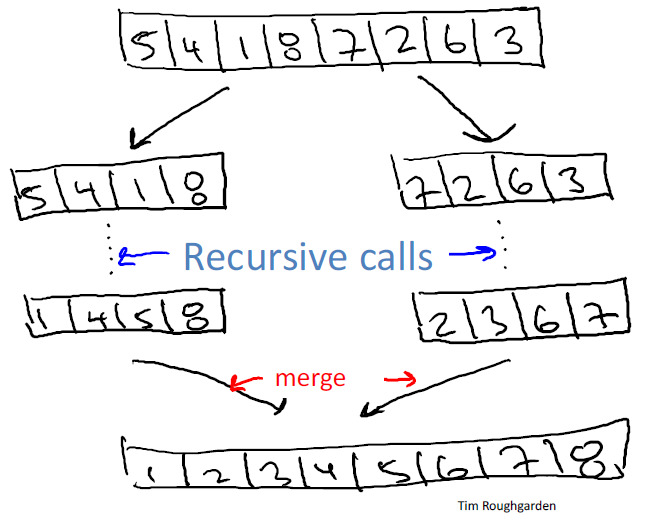Ordinal Data - Merge sort Algorithm

John von Neumann invented merge sort in 1945. It requires O(n log n) comparisons. A sorting algorithm for rearranging lists (or any other data structure that can only be accessed sequentially, e.g. file...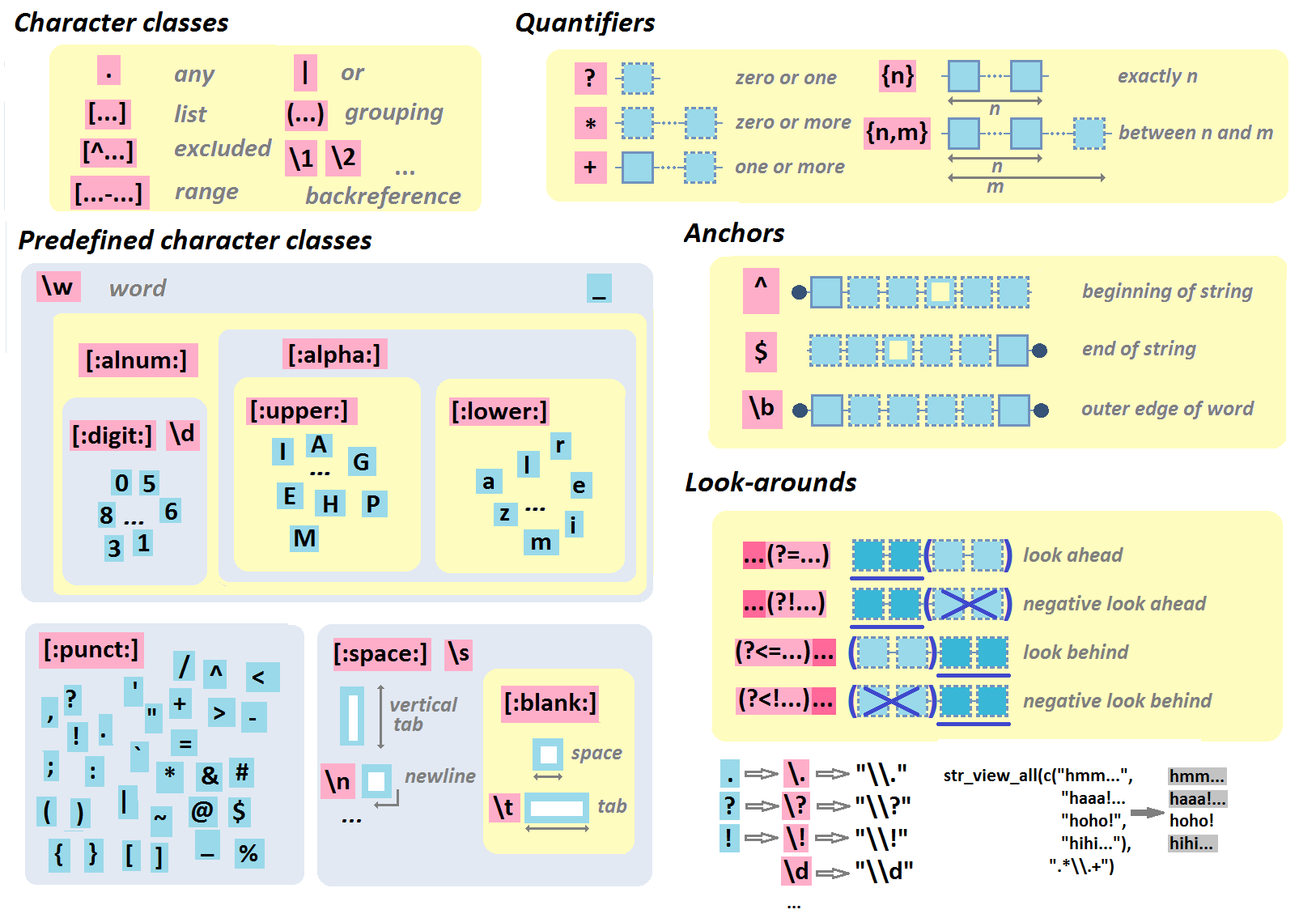Recursion

? define the group name for the expression enclosed in parenthesis. ie (A \g defines that the expression should be found (defined by the greedy quantifier) A \g defines a pattern that: =====...